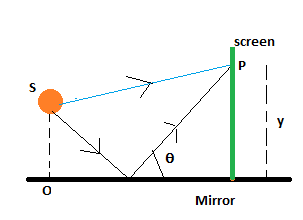# Interference effects are produced at point P on a screen as a result of direct rays from a 470 nm...

## Question:

Interference effects are produced at point P on a screen as a result of direct rays from a 470 nm source and reflected rays off a mirror, as in figure below. If the source is 130 m to the left of the screen and 1.50 cm above the mirror, find the distance y (in millimeters) to the first dark band above the mirror.## Constructive and Destructive Interference:

When two waves meet in such a way that their crests line up together, then it's called constructive interference. The resulting wave has a higher amplitude. In destructive interference, the crest of one wave meets the trough of another, and the result is a lower total amplitude.

## Answer and Explanation:

The wavelength of light from the source:

{eq}\lambda = 470 nm {/eq}

Distance from source to the screen: L= 130 m

The source is at a distance d1 = 1.50cm from the mirror

Here the direct ray of light does not undergo any phase change

whereas the reflected ray of light has suffered a phase change of 180 degrees

so the conditions for constructive and destructive interference are reversed

The dark bands occur at a distance of

{eq}y= \frac{m \lambda L}{d} {/eq}

The first dark band m=1 and at a distance of

{eq}y= \frac{1* 470*10^-3 * 130}{1.50*10^-2} {/eq}

= 4.07*10^-3 m

=4.07 mm